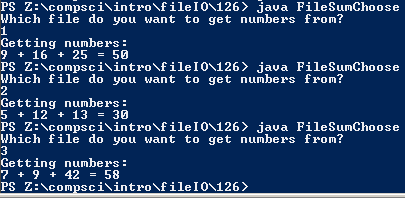# Assignment #125: Summing Three Numbers from Any File

## Code

```import java.io.File;
import java.util.Scanner;

public class FileSumChoose {

public static void main(String[] args) throws Exception {

Scanner input1 = new Scanner(new File("Nums1.txt"));
Scanner input2 = new Scanner(new File("Nums2.txt"));
Scanner input3 = new Scanner(new File("Nums3.txt"));
Scanner kb = new Scanner(System.in);

int a = 0, b = 0, c = 0, file;

System.out.println("Which file do you want to get numbers from?");
file = kb.nextInt();

if (file == 1) {

System.out.println("Getting numbers: ");
a = input1.nextInt();
b = input1.nextInt();
c = input1.nextInt();

}

if (file == 2) {

System.out.println("Getting numbers: ");
a = input2.nextInt();
b = input2.nextInt();
c = input2.nextInt();

}

if (file == 3) {

System.out.println("Getting numbers: ");
a = input3.nextInt();
b = input3.nextInt();
c = input3.nextInt();

}

System.out.println(a + " + " + b + " + " + c + " = " + (a + b + c));

}

}
```

### Picture of the output